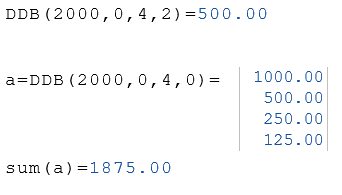# Online calculator - DDB function

Online calculator returns the depreciation of an asset for a specific time period

## Description of the function DDB

Returns a value specifying the depreciation of an asset for a specific time period using the double-declining balance method or some other method you specify.

The double-declining balance method computes depreciation at an accelerated rate. Depreciation is highest in the first period and decreases in successive periods.

The declining balance depreciation method is an accelerated depreciation, the higher depreciation amounts in the first few years of the useful life of an asset and greater tax savings than the straight-line amortization method, where the amounts are always the same.

The Life and Period arguments must be expressed in the same units. For example, if Life is given in months, Period must also be given in months. All arguments must be positive numbers.

## Calculate depreciation online

Cost
Initial cost
Worth
Worth at end of life
Life
Length of useful life
Period
Period of depreciation
Factor
Rate of declines

Decimal places

### Result

Depreciation in period

## Description of the parameters

Cost

The initial cost of the asset.

Worth

The value of the asset at the end of its useful life.

Life

The length of useful life of the asset.

Period

The period for which asset depreciation is calculated.

Factor

The rate at which the balance declines. The default is 2 (double-declining method).

## RedCrab Calculator

In the RedCrab Calculator , you use the DDB function for calculation of the depreciation of an asset for a single period or all periods.

### Example